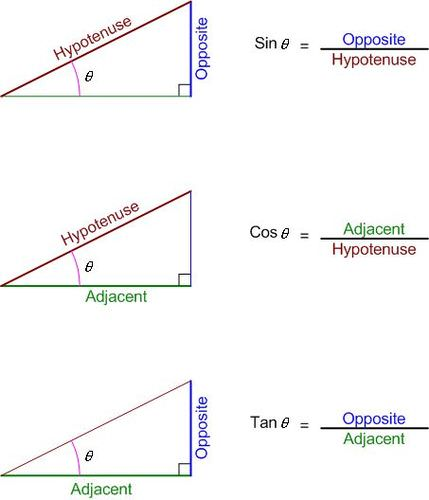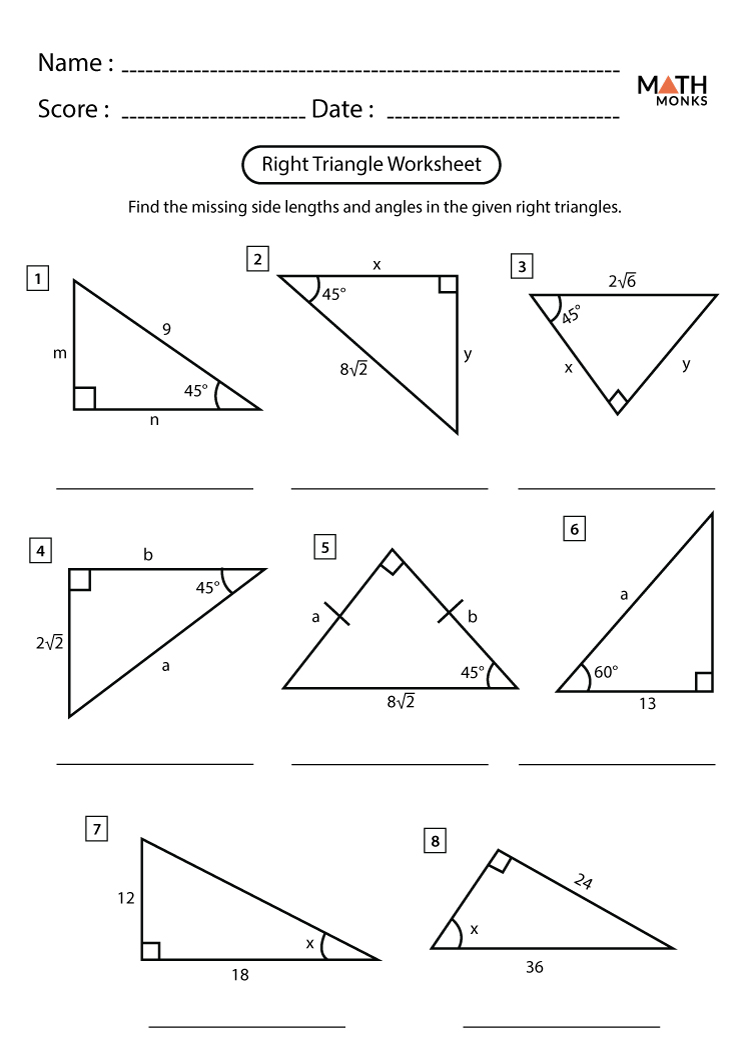# TRIGONOMETRIC RATIOS: Sine, Cosine and Tangent

Introduction

Lengths, angles, triangles, circles, and all shapes play a major role in our lives, whether we realise it or not. Mathematics, as such, makes use of this more often than not.

Today, we will zoom in onto triangles, taking a deeper look at their angles and side lengths. In order for us to get a better understanding of trigonometry before we delve deeper into the topic of today's lesson, let's watch the following video:

https://youtu.be/vbhahuTuMo4

Now that we've covered the basics of trigonometry and we know that we are dealing with right-angled triangles, let's look at the 3 basic trigonometric ratios.So an easy way for us to remember these ratios is by remembering that the schoolboys went on a Soh Cah Toa (Soccer tour), where S is for sin, o is for opposite, h is the hypotenuse, C is for Cos, a is for adjacent and T is for Tan.

For this task, you will solve a few problems that deal with trigonometric ratios. This task will assist you to better understand the concepts and apply them to problem-solving. Use the resources provided to you to guide you when attempting the task. You may use external resources such as your textbook if it will help you understand the topic better. Have fun and good luck!Resources:

https://www.texasgateway.org/resource/applying-trigonometric-ratios

https://physics.info/trigonometry/summary.shtml

Process

• Read through the Introduction and make use of all resource links to videos and books provided, to complete the task.
• Familiarise yourself with the different ratios and when to use which ratio so that it makes answering the task easier.
• Answer all the questions and provide only the answers to the sides/angles marked with a letter of the alphabet.
• Make use of a calculator as far as possible and round off answers to 1 decimal place.
• Keep any rough work on the side.
• The theorem of Pythagoras may be used to answer some questions.
• If you've used a ratio to find one side and the theorem of Pythagoras to find the other, you will not lose any marks.
• The following video will assist you with the use of a calculator:

• For more information on the application of the ratios, you may attain information on your own or the following resources may be used:

Evaluation

Rubric for Assessing the Mathematics task on right-angled triangles

 QUESTION Ratio used (side lengths) Ratio used (angle) Substitution (side lengths) Substitution (angle) Answer   (side lengths) Answer (angle) TOTAL 1 2 (1 per side) - 2 (1 per side) - 2 (1 per side) - 6 2 2 (1 per side) - 2 (1 per side) - 2 (1 per side) - 6 3 2 (1 per side) - 2 (1 per side) - 2 (1 per side) - 6 4 2 (1 per side) - 2 (1 per side) - 2 (1 per side) - 6 5 2 (1 per side) - 2 (1 per side) - 2 (1 per side) - 6 6 1 - 1 - 1 - 3 7 - 1 - 1 - 1 3 8 - 1 - 1 - 1 3

1 mark for attempting/completing the entire task

TOTAL MARKS = 40

Conclusion

This lesson dealt with an introduction to trigonometry and the 3 basic trigonometric ratios.

We saw the application of these ratios to real-life situations and we familiarised ourselves with the different ratios and its' application to find missing lengths and angles.

The task assisted us in understanding and applying the different concepts. The task consisted of 40 marks and will be used as a summative assessment.

In preparation for the next lesson, please watch the following video:

Credits

All videos in this lesson are obtained from Youtube.

Links to any books were also obtained using the google search engine and is an online version. A hard copy of the same textbook could be used.

If you wish to know some more on trigonometry as a whole, you may watch this video:

Teacher Page

Maryam Solarie

215048660

Cape Peninsula University of Technology - PGCE: FET course